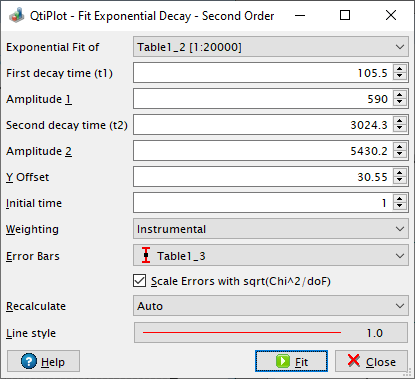# Fit Exponential Decay - Second Order

This dialog box is opened when you select the Fit Exponential Decay -> Second Order... command from the Analysis -> Fitting menu. It allows to choose the curve to fit, the initial guesses for the fit parameters and the weighting method.

Figure 5-113. The Fit Exponential Decay Second Order dialog box.The two exponentials decay fit uses the following equation:

y = Y0 + A1e(-x/t1) + A2e(-x/t2)

where Y0 corresponds to the Y Offset, t1 is the First decay time and t2 the Second decay time.

The Initial time represents the left abscissa limit for the fit data. QtiPlot guesses initial values for these parameters upon selection of the curve to fit, but it might be necessary to modify them in order for the fit to converge.

The available weighting methods are:

1. No weighting: all weighting coefficients are set to 1 (wi = 1).

2. Instrumental: the values of the associated error bars are used as weighting coefficients wi = 1/eri2, where eri are the error bar sizes stored in error bar columns. You must add error bars to the analyzed curve before performing the fit.

3. Statistical: the weighting coefficients are calculated as wi = 1/yi, where yi are the y values in the fitted data set.

4. Direct Weighting: the weighting coefficients are calculated as wi = yi, where yi are the y values in the fitted data set.

If the curve selected for the data fit operation has error bars attached, the weighting method is automatically set to Instrumental and a list box displays the names of the available error bar curves.

It is also possible to specify how the fit operation responds to modifications of the source data curve using the Recalculate list box. The available options are:

No

- No recalculation when data changes, the fit object is deleted from memory when the operation ends.

Auto

- A recalculation is automatically performed when input data changes.

Manual

- No recalculation is triggered when data changes, instead you can perform a recalculation at any time by pressing the Recalculate button from the Analysis tab of the plot details dialog.

The recalculation mode can be changed later on via the Analysis tab of the plot details dialog.